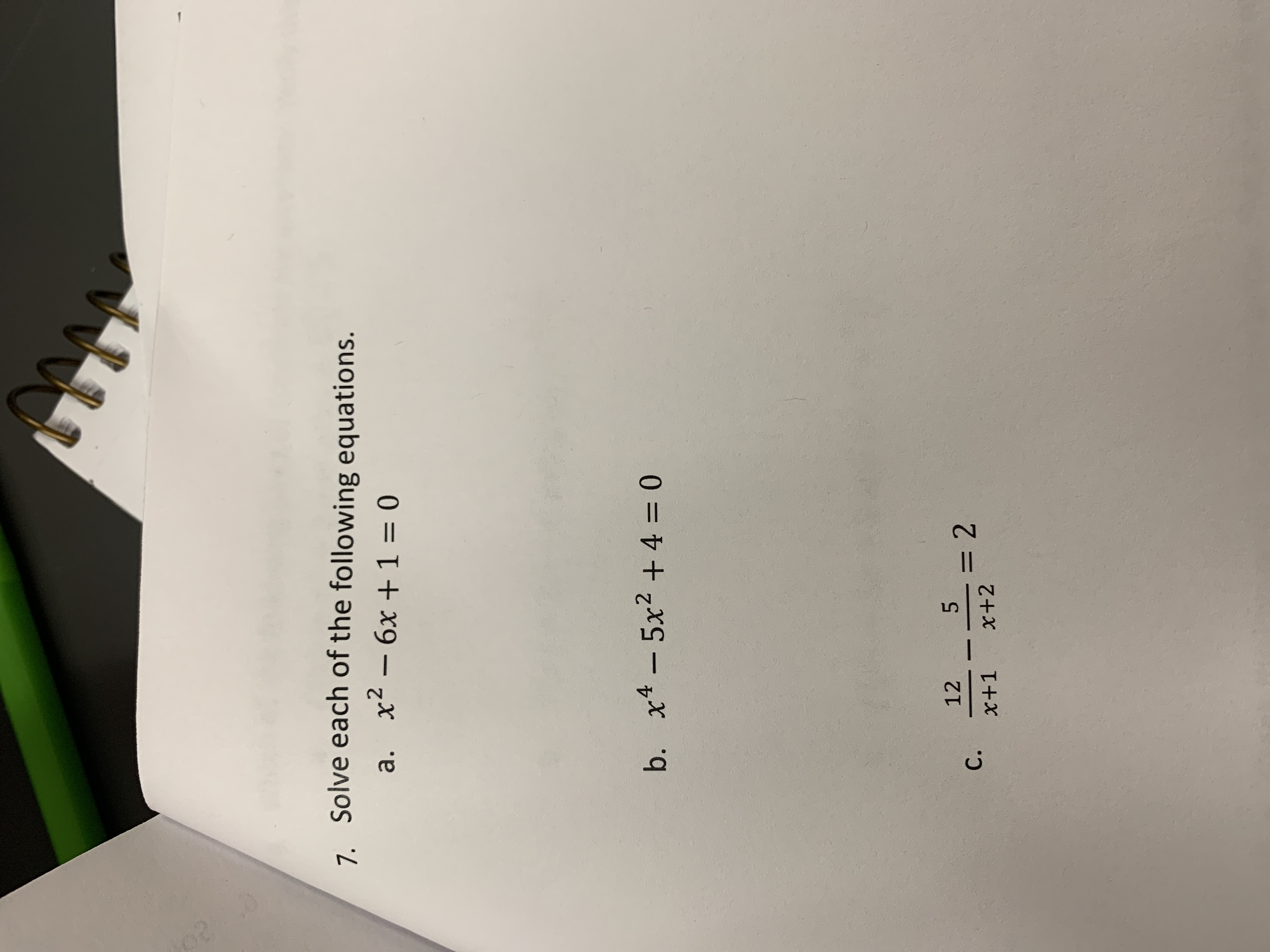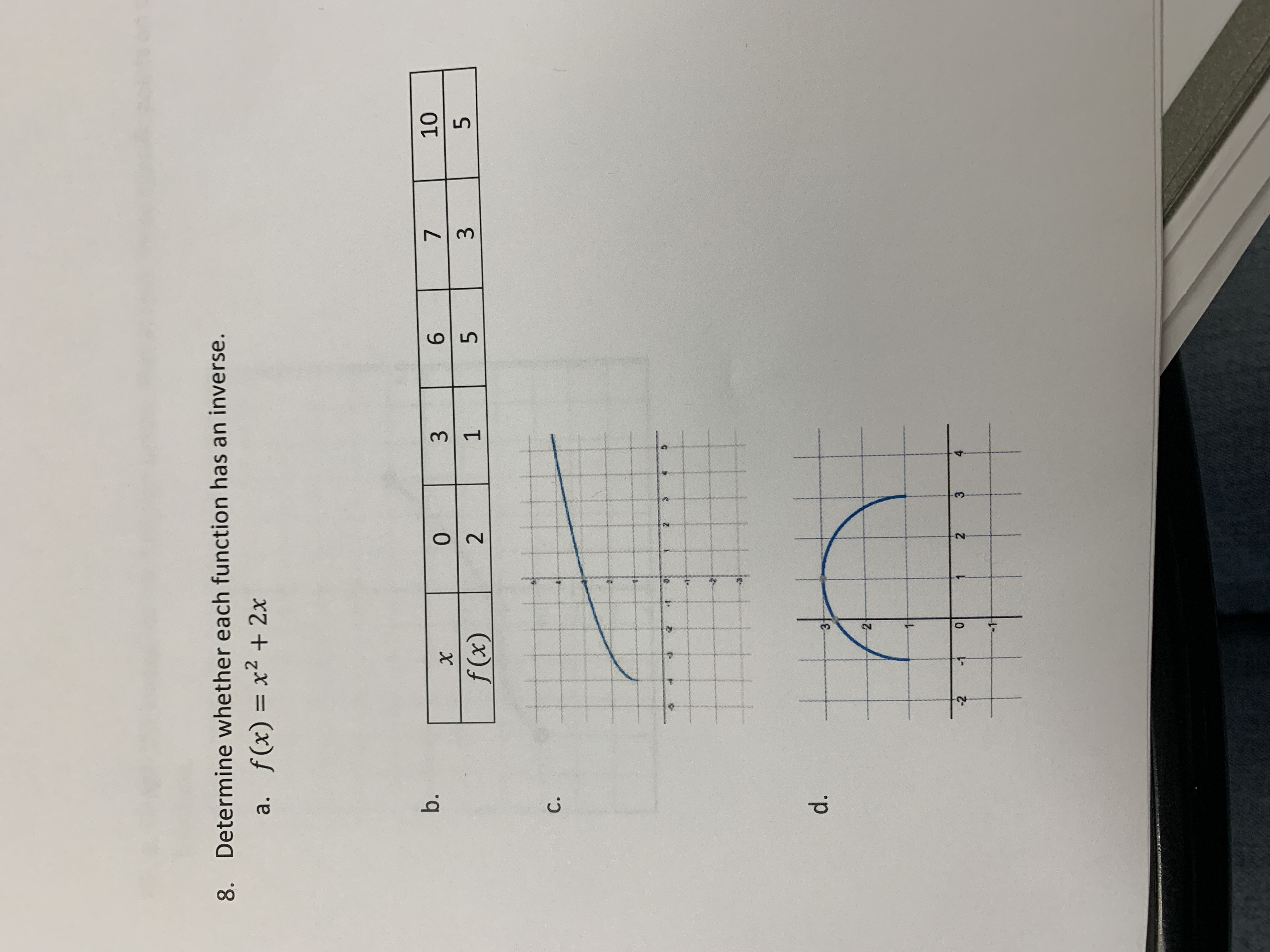# 7. Solve each of the following equations. a. x2- 6x +1 = 0 b. x4-5x2 +4 = 0 5 = 2 x+2 12 С. x+1 1 02 Determine whether each function has an inverse. 8. a. f(x) = x2 + 2x 10 b. 7 0 X 5 3 1 f (x) 2 C. d. 2- 4 3 0 2 -2 -1 6 Ln 3

Questionhelp_outlineImage Transcriptionclose7. Solve each of the following equations. a. x2- 6x +1 = 0 b. x4-5x2 +4 = 0 5 = 2 x+2 12 С. x+1 1 02 fullscreenhelp_outlineImage TranscriptioncloseDetermine whether each function has an inverse. 8. a. f(x) = x2 + 2x 10 b. 7 0 X 5 3 1 f (x) 2 C. d. 2- 4 3 0 2 -2 -1 6 Ln 3 fullscreen

### Want to see this answer and more?

Experts are waiting 24/7 to provide step-by-step solutions in as fast as 30 minutes!*